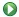1 A First Look

2 Assigning to a Tuple

Prev:  Session 1

Next:  Session 3

Contents

Session 2   Some Trivial Algorithms

Leslie Lamport

1  A First Look at Algorithms

We will now begin looking at PlusCal algorithms.  The tutorial describes most of the PlusCal language, but you might want to download and save the PlusCal manual. It contains a few features that aren't described here and provides more detailed descriptions of ones that are.

Our first algorithm is in specification Session2.  Open it in the Toolbox the same way you opened the specification Session1 in Section 2.1 of Session 1 .

This time, pay attention to how the module begins and ends.  (Beginners sometimes forget to add the MODULE line or put their specification after the end of the module, where it's ignored.)  Again, ignore the EXTENDS statement.  The algorithm is contained in a comment, which is enclosed by the delimiters (* and *).  (You can also put such comments inside the algorithm.)  The algorithm itself begins with:

--algorithm AnyName {
where AnyName can be replaced by any TLA+ identifier That name is not used anywhere.  The algorithm is terminated by a matching right brace } .

The body of the algorithm begins with a declaration of all its variables.  The statement

variable x = 1 ;
declares x to be the only variable and to have the initial value 1.  The rest of the algorithm's body is code enclosed in curly braces { }.  In this example, that code simply adds 1 to x and then prints the value of x:

x := x + 1 ;
print x
Unlike most of today's popular programming languages, PlusCal uses  :=  for assignment.  It uses  =  to mean what it's meant for hundreds of years.  As in many languages, the semicolon ; indicates that the print statement is to be executed immediately after the assignment statement.  Semicolons must come between statements, but you can put a semicolon after the print statement if it makes you happier.

To execute the algorithm, we must first run the PlusCal to TLA+ translator.  You can run the Translate PlusCal Algorithm command from the Toolbox's File menu, but it's much easier to just type Control-T.  This will add the resulting TLA+ specification to the module right below the comment containing the algorithm.  Don't try to read that specification.

As you've seen, TLC is run on a model.  This time, you will create a new model.  As before, click on TLC Model Checker in the Toolbox's top menu bar, but this time choose  New Model . This raises the New model window that allows you to choose a name for the model.  I'm usually happy with the default name Model_1.  Clicking OK opens the Model Overview sub-tab of the new model.  Ignore what's on it.  Run TLC by clicking on the iconin the upper-left corner of the sub-tab.  TLC should run for a few seconds and then raise the model's Model Checking Results sub-tab.  The output of the print statement appears in the sub-tab's User Output section.  It obviously should show the value 2.

PlusCal treats all mathematical values and operations on them the same, which is the way most programming languages treat only numbers and a handful of other kinds of values.  Check this out by doing the following exercise.

Exercise 1   Modify algorithm AnyName to give x the set {"a","b"} as its initial value and so the assignment statement assigns to x the set obtained by adding to this set the string "c".  (If you don't remember how to add an element to a set, review the \cup set operation in Section 2.5 of Session 1 .)  Save the module, run the translator, and then run TLC (on the same model) to check that it prints {"a", "b", "c"} Show the answer.

--algorithm AnyName {
variable x = {"a","b"} ;
{
x := x \cup {"c"} ;
print x
}
}

Exercise 2   Now modify the algorithm so that instead of adding "c" to the set {"a", "b"}, it removes the "a" from that set.  Show the answer.

--algorithm AnyName {
variable x = {"a","b"} ;
{
x := x \ {"a"} ;
print x ;
}
}

2  Assigning to a Tuple

Now let's try setting x to a tuple and modifying it.  Enter the following algorithm body, which you can do by typing or copy/pasting.

variable x = <<1, 2, 3>> ;
{
x := x + 4 ;
print x
}

Save the module, run the translator, and run TLC.  It should print <<1, 2, 6>>, which is probably what you expected.

Now modify the algorithm by adding the green code shown here:

variable x = <<1, 2, 3>> , y = x ;
{
x := x + 4 ;
print x  ;
print y
}
This declares a second variable y (note the comma between the two variable declarations) and declares its initial value to be the initial value of x, which is <<1, 2, 3>>.  This is legal because x has already been declared and its initial value specified.  The code prints the value of y after it prints the value of x.  It will, of course, print the same value of x as before.  What value do you expect it to print for y?  Save/translate/run TLC and see what it actually prints.

Were you surprised?  You shouldn't have been.  PlusCal treats all mathematical values the same.  If the algorithm had initialized x and y to the number 42, you wouldn't have expected assigning a new value to x to have changed the value of y.  Since all values are handled the same, this is true for assigning a tuple to the variables.  So, you should have expected the print y statement to have printed <<1, 2, 3>>, the initial value of y.  If that's not what you expected, click here.